×#### Thank you for registering.

One of our academic counsellors will contact you within 1 working day.

Click to Chat

1800-1023-196

+91-120-4616500

CART 0

• 0

MY CART (5)

Use Coupon: CART20 and get 20% off on all online Study Material

ITEM
DETAILS
MRP
DISCOUNT
FINAL PRICE
Total Price: Rs.

There are no items in this cart.
Continue Shopping```Practical Problems on Union and Intersection of Two Sets

Table of Content

Union of Sets

Union of two Joint Sets

Union of two Disjoint Sets

Intersection of Sets

Difference of two Sets

Union and Intersection of three Sets

Complement of a Set

Problems related to Union and Intersection of Sets

To solve the practical problems related to union and intersection, first we need to summarize the meaning of union, intersection and difference of sets. And understand the formulas related to them.

Union of Sets

The Union of two sets A and B is the set of all the elements present in A or B or both. We will take the common elements at once only. It is denoted with the symbol “∪” read as “union”.

Symbolically,

A∪B = {x: x ∈A or x ∈B}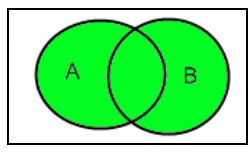Union of two Joint Sets

If A and B are two finite sets and there is some common elements within them, that is, A∩B≠∅

Then,

n (A∪B) =n (A) +n (B)-n (A∩B)

Union of two Disjoint SetsIf A and B are two finite sets and there is no common elements within them, that is, A∩B=∅

Then,

n (A∪B) =n (A) +n (B)

Intersection of Sets

The intersection of two sets A and B means a set of all the elements which are common to both A and B. It is represented by symbol “∩” reads as “Intersection”.

Symbolically,

A∩B = {x: x ∈ A and x ∈ B}Difference of two Sets

The difference of two Sets A and B represents as A-B that is, all the element which are present in A but not in B.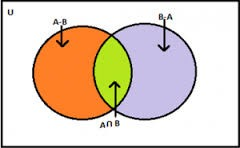Symbolically,

A – B = {x: x ∈ A and x ∉ B} and vice versa.

A-B is not necessarily equal to B-A

The above figure shows that the sets A – B, A ∩ B and B – A are disjoint sets, it means, the intersection of any of these two sets is the empty set that is, ∅

So,

n (A∪B) = n (A-B) + n (A∩B) + n (B-A)Union and Intersection of three Sets

If A, B and C are three finite joint sets, then their union will be,

n (A ∪ B ∪ C) = n (A) + n (B) + n (C) - n (A ∩ B) – n (A ∩ C) - n (B ∩ C) + n (A ∩ B ∩ C)

Complement of a Set

The complement of a set A is the set of all the elements which are the elements of Universal set but not the elements of A. It is represented by symbol A′ or Ac.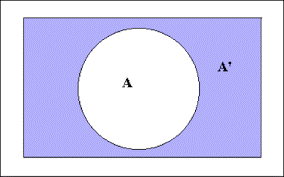Symbolically,

A′ = {x: x U, x A}

A′=U-A

We must remember some properties of complement of sets to solve the problems related to it.

Properties of Complement of Sets are:

Difference Laws

A-(B∪C) = (A-B) ∩ (A-C).

A-(B∩C) = (A-B) ∪ (A-C)

De Morgan’s Law

(A ∪ B)′ = A′ ∩ B′

(A ∩ B)′ = A′ ∪ B′

Problems related to Union and Intersection of Sets

Example 1

There are 500 students in a school, 220 like science subject, 180 like math and 40 like both science and math. Find the number of students who like

Science but not math

Math but not science

Either math or science

Solution

Let the total number of students be U that is, the universal set.

Let A is the set of number of students who like science.

Let B is the set of number of students who like math.

Here, n (U) =500      n (A) =220    n (B) =180    n (A∩B) =40Here we have to find the number of students who like science but not math, so symbolically we have to find A-B.

As it is showing in the Venn diagram,

A = (A-B) ∪ (A∩B)

n (A) = n (A-B) + n (A∩B)

n (A-B) = n (A) - n (A∩B)

= 220 – 40 = 180

Hence, the numbers of students who like science only not math are 180.

Here we have to find the number of students who like math but not science, so symbolically we have to find B-A.

B = (B-A) ∪ (A∩B)

n (B) = n (B-A) +n (A∩B)

n (B-A) =n (B)-n (A∩B)

=180-40=140

Hence, the numbers of students who like math only not science are 140.

Here we have to find the number of students who like either math or science.

n (A∪B) = n (A) + n (B) – n (A∩B)

= 220 + 180 – 40

= 360

Example 2

In a college, there are 20 students who enrolled for commerce only,90 students enrolled for mathematics only, 30 students enrolled for commerce and mathematics both and 60 students enrolled for others. Find

The total number of students in college

Total number of students enrolled either for commerce or mathematics

Total number of students enrolled for commerce

Total number of students enrolled for mathematics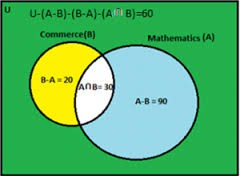Solution

Given in the Venn diagram,

Let total number of students as U

Students of Commerce as B

Students of Mathematics as A

B – A = 20        A – B = 90           A∩B = 30

As it is given in Venn diagram,

n (U)-n (A-B)-n (B-A)-n (A∩B) = 60

n (U) = 60+ n (A-B) + n (B-A) + n (A∩B)

= 60 + 90 + 20 + 30

= 200

Hence there are total 200 students in college.

n(A∪B) = n(A-B) + n(A∩B) + n(B-A) that is, the formula of difference of sets

= 90 + 30 + 20

= 140

Hence there are 140 students who enrolled either for commerce or mathematics.

n(B) = n(B-A) + n(A∩B)

= 20 + 30

= 50

Hence there are 50 students who enrolled for commerce.

n(A) = n(A-B) + n(A∩B)

= 90 + 30

= 120

Hence there are 120 students who enrolled for mathematics.

Example 3

In a shop, 380 people buy socks, 150 people buy shoes and 200 people buy belt. If there are total 580 people who bought either socks or shoes or belt and only 30 people bought all the three things? So how many people bought exactly two things.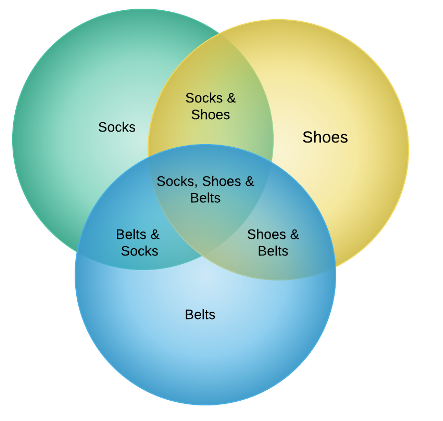Solution

Let S, H and B represent the set of number of people bought socks, shoes and belt respectively.

So, n(S) = 380, n (H) = 150, n (B) = 200

n (S∪H∪B) =580, n (S∩H∩B) =30

Therefore, n (S ∪ H ∪ B ) = n ( S ) + n ( H )+ n ( B ) – n (S ∩ H ) – n (H ∩ B ) – n (B ∩ S ) +  n ( S ∩ H ∩ B ),

Now, we will put values given in the formula,

580 = 380 + 150 + 200 - n (S ∩ H) – n (H ∩ B) – n (B ∩ S) + 30

This gives that,

n (S ∩ H) + n (H ∩ B) + n (B ∩ S) =180

But this includes the number of people who bought all the three items also. So we have to deduct these numbers of people from it.

Let, n (S ∩ H ∩ B) = a

As we can see from the Venn diagram,

n (S ∩ H)-a + n (H ∩ B)-a + n (B ∩ S)-a=the required number

n (S ∩ H) + n (H ∩ B) + n (B ∩ S)-3a

180 – 90 = 90

Hence, 90 people are there who bought exactly two things.

Example 4

In a school there are 25 teachers who teach engineering or management. Of these, 15 teach engineering and 6 teach both engineering and management. How many teach management?Solution

Let M be the set of teachers who teach management and E be the set of teachers who teach engineering. Here ‘or’ means union and ‘and’ means intersection. So, we have

n ( E ∪ M ) = 25 , n ( E ) = 15 and n ( E ∩ M ) = 6

We have to calculate n ( M ).

According to formula

n ( E ∪ M ) = n ( E ) + n ( M ) – n ( E∩ M ),

We obtain

25 = 15 + n ( M ) – 6

Thus n ( M ) = 16

Hence 16 teachers teach management.

Example 5

If there are 400 elements in consideration, set A has 100 elements, set B has 150elments, and 75 are the common elements. Find how many elements is neither in A nor in B.Draw the Venn diagram for supporting your answer.

Solution

Let U denote the set of total elements.

Given

n (U) = 400, n (A) = 100, n (B) = 150 and n (A ∩ B) = 75.

We have to calculate the elements which are neither in A nor in B and according to the De Morgan’s law of complement law, we know that

n (A′ ∩ B′) = n (A ∪ B)′

= n (U) – n (A ∪ B) i.e. the complement of any set is U –A and here we have to calculate the complement of the union of A and B so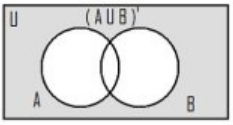n (A ∪ B)′= n (U) – n (A ∪ B)

= n (U) – n (A) – n (B) + n (A ∩ B)

= 400 – 100 – 150 + 75 = 225

Hence the number of elements neither in A nor in B are 225.

The Venn diagram of the n (A ∪ B)′ is shown on the right figure:

Example 6

There was an investigation of 500 families having pet.400 were having dog as pet and 200 were have cat as pet, 50 owned both cat and dog as pet. Now find if this data is correct? Justify your answer.

Solution

Let U be the set of families investigated, D be the set of families having dog as pet and C be the set of families having cat as a pet.

Given,

n ( U ) = 500, n (D ) = 400, n ( C ) = 200 and n ( D ∩ C ) = 50.

Now we will put the values in the formula of union of two sets.

n ( D ∪ C ) = n ( D ) + n ( C ) – n ( D ∩ C )

= 200 + 400 – 50

= 550

Here the union of two sets is 550 and the total numbers of families investigated are 500.

D ∪ C ⊂ U implies n ( D ∪ C) ≤ n ( U ).

The union of two sets should be the subset of universal set that is, it should be less than or equal to universal set.

As there is a contradiction, so, the given data is incorrect.

Watch this Video for more reference

Practical Problems on Union and Intersection of Two Set
```### Course Features

• 731 Video Lectures
• Revision Notes
• Previous Year Papers
• Mind Map
• Study Planner
• NCERT Solutions
• Discussion Forum
• Test paper with Video Solution